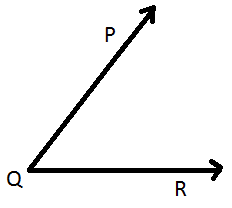## Shapes and Designs/ Two dimensional Geometry

Angles two rays with a common endpoint, vertex, measured in degrees. Rotates counter-clockwise. Name by starting ray, vertex, ending ray.angle RQP

Supplementary angles are two angles that form a straight line, the sum is 180 degrees

Complimentary angles are two angles that form a right angle, the sum is 90 degrees

Triangles
are described by their sides and angles. An angle that is less than 90 degrees is acute, one that is exactly 90 degrees is right and one that is greater than 90 is obtuse. The sides are all equal it is equilateral, if two sides are equal it is isosceles and if none are equal it is scalene.

For example: Describe a triangle the angles of 90, 45 and 45 and sides of 5 cm, 5 cm and 7 cm.  That is a right isosceles triangle.

Triangles may be drawn when the sum of two sides is greater than the third.

The minimum information about a triangle that allows you to draw exactly on triangle is angle-side-angle, side-side-side or angle-angle-side.

Rhombus has four equal sides opposite sides parallel and opposite angles equal.

Square had four equal sides and all 90 degree angles.

Rectangle has opposite sides parallel and all 90 degree angles.

Trapezoid has exactly one pair of parallel sides.

Parallelogram has opposite sides are parallel and opposite angles are equal.

Quadrilaterals may be drawn when the sum of the three shorter sides is greater than the largest side.

Rotation

Rotation angles are measured from 0 degrees to 360 degrees or more.

Measure rotation angles in  a counterclockwise direction.

Initial side is the ray showing the starting direction,

Terminal side is the ray showing the ending direction.

Polygons

Sum of the angles = 180n - 369 or 180(n-2)  when n = number of sides

Measure of an angle = sum/n or 180(n-2)/n

Convex Polygons is a polygon with all interior angles measuring less than 180 .

Concave Polygons, like arrow and star, has at least one interior angle that measures greater than 180.

Interior Angle is an angle inside the polygon formed by two adjacent sides.

Exterior Angle is formed by extending a side, the extension and the other side meet at the vertex.

Angles

In a parallelogram, opposite angles are equal and consecutive angles are supplemental.

Vertical angles, opposite angles when parallel lines are bisected by a transversal, are congruent, equal.

Constant of Proportionality

constant of proportionality (K=y/x)